Anúncio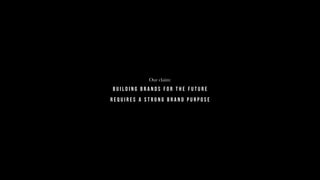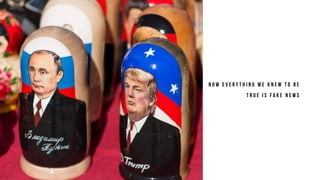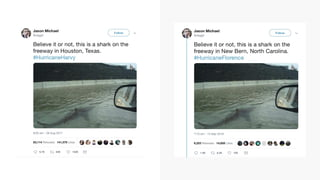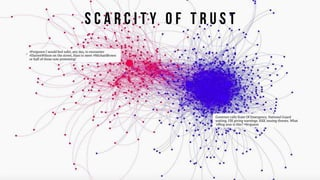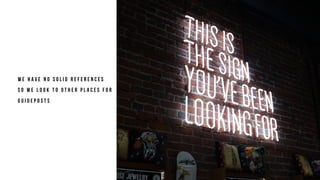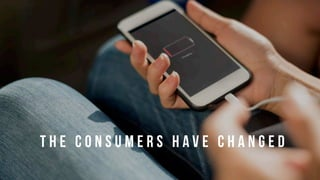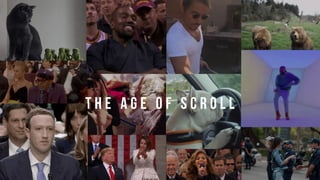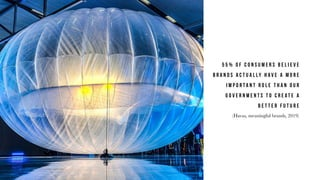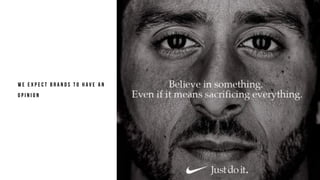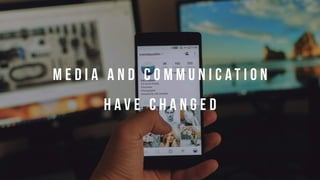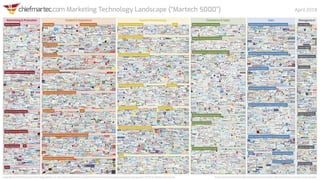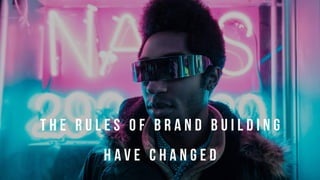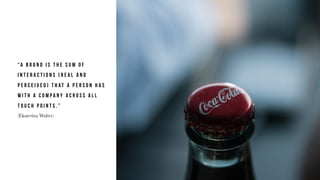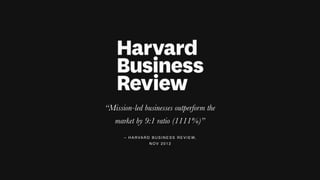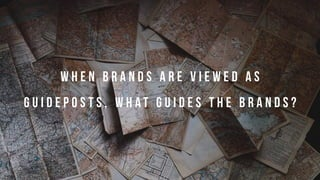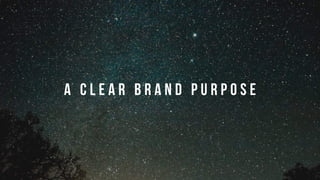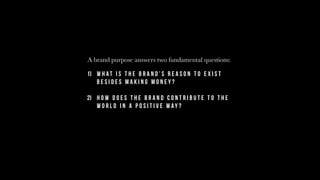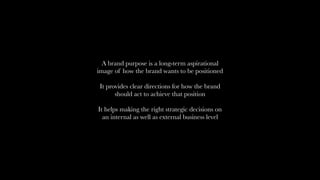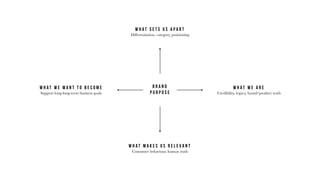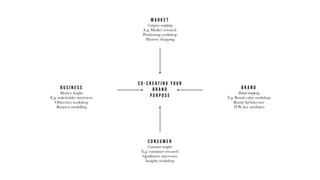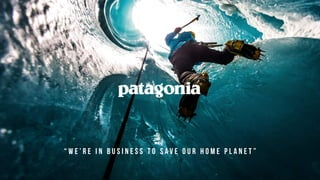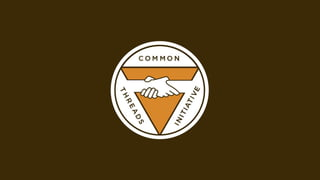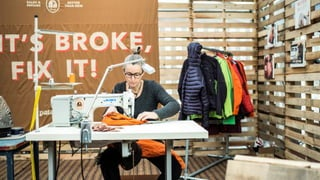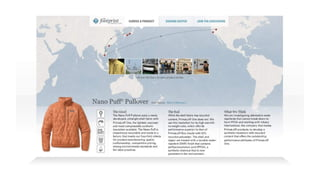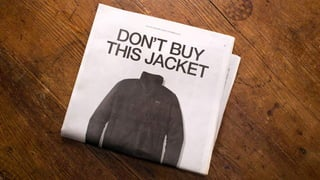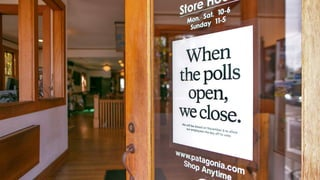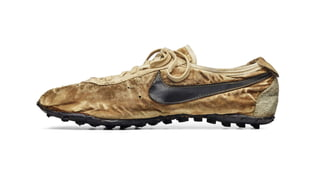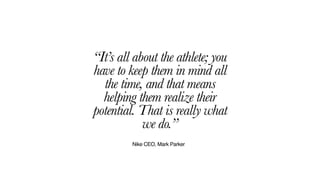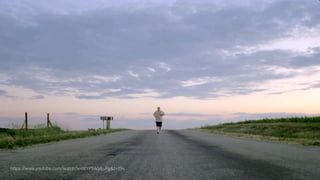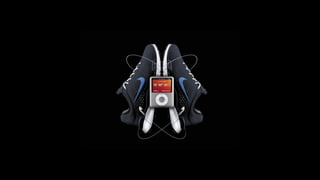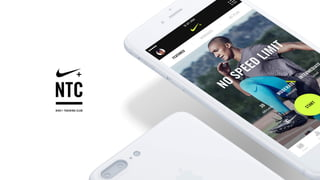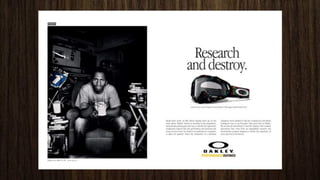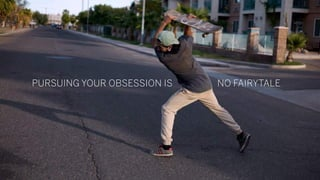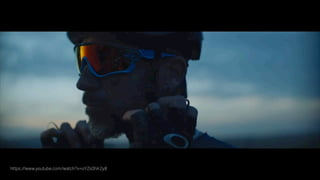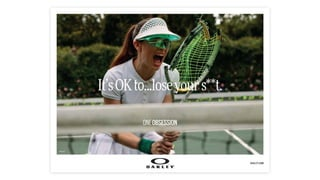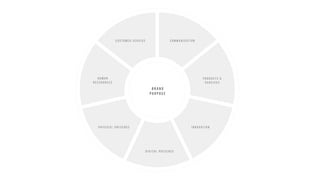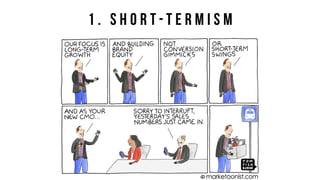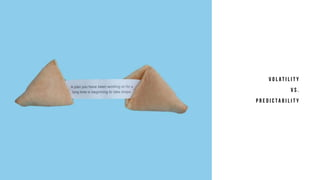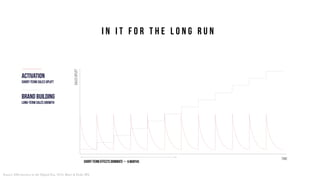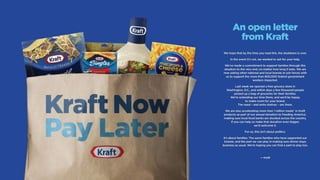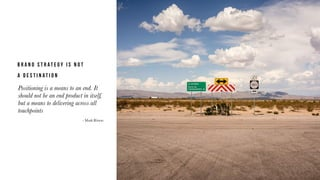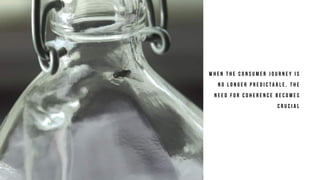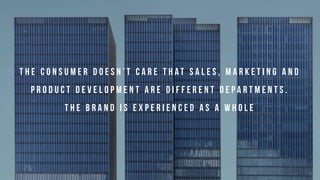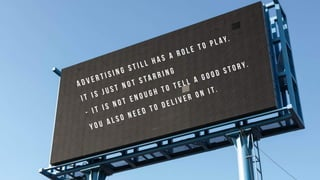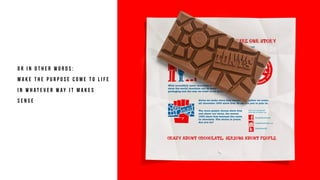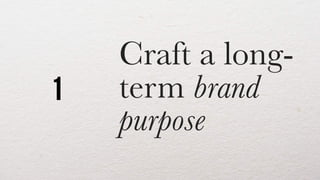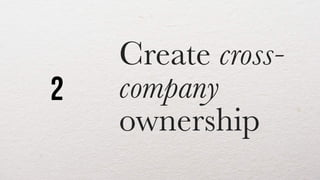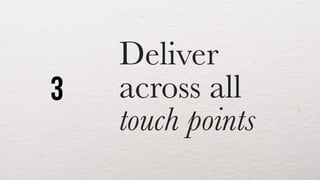1 de 78
Anúncio

### Purposeful Brands

1. P u r p o s e f u l b r a n d s Miriam Plon Sauer Strategy director miriam.plon@akqa.com © AKQA
2. W E E X I S T T O C R E A T E T H E F U T U R E W I T H O U R C L I E N T S .
3. Our claim: B U I L D I N G B R A N D S F O R T H E F U T U R E R E Q U I R E S A S T R O N G B R A N D P U R P O S E
4. This talk: W H Y B R A N D P U R P O S E H O W D O E S I T W O R K T H E M O S T C O M M O N T R A P S H O W T O O V E R C O M E T H E M
5. T H E W O R L D H A S C H A N G E D
6. W E U S E D T O H A V E R E L I G I O N , T R A D I T I O N A N D A U T H O R I T I E S W E C O U L D T R U S T
7. N O W E V E R Y T H I N G W E K N E W T O B E T R U E I S F A K E N E W S
8. S C A R C I T Y O F T R U S T
9. W E H A V E N O S O L I D R E F E R E N C E S S O W E L O O K T O O T H E R P L A C E S F O R G U I D E P O S T S
10. T H E C O N S U M E R S H A V E C H A N G E D
11. I W W I W W I W I
12. T H E A G E O F S C R O L L
13. T H E E X P E C T A T I O N E C O N O M Y
15. B R A N D S A R E T H E N E W B A N D S
16. 5 5 % O F C O N S U M E R S B E L I E V E B R A N D S A C T U A L LY H A V E A M O R E I M P O R T A N T R O L E T H A N O U R G O V E R N M E N T S T O C R E A T E A B E T T E R F U T U R E (Havas, meaningful brands, 2019)
17. W E E X P E C T B R A N D S T O H A V E A N O P I N I O N
18. M E D I A A N D C O M M U N I C A T I O N H A V E C H A N G E D
19. E V E R Y 1 0 M I N U T E S , W E C R E A T E A S M U C H I N F O R M A T I O N A S T H E F I R S T 1 0 , 0 0 0 G E N E R A T I O N S O F H U M A N B E I N G S
20. T H E P O I N T O F E N G A G E M E N T A N D T H E P O I N T O F S A L E S A R E M E LT I N G T O G E T H E R
21. F R O M L I N E A R L O G I C A L C O N S U M E R J O U R N E Y T O ‘ F LY I N A B O T T L E ’
22. T H E R U L E S O F B R A N D B U I L D I N G H A V E C H A N G E D
23. W H A T D O E S I T T A K E T O B U I L D B R A N D S F O R T H E F U T U R E ?
24. “A B R A N D I S T H E S U M O F I N T E R A C T I O N S ( R E A L A N D P E R C E I V E D ) T H A T A P E R S O N H A S W I T H A C O M P A N Y A C R O S S A L L T O U C H P O I N T S . ” (Ekaterina Walter)
25. A B R A N D I S E S S E N T I A L LY A C O N N E C T I O N . I T S H O U L D B E P E R S O N A L
26. S T A N D F O R S O M E T H I N G
27. “Mission-led businesses outperform the market by 9:1 ratio (1111%)” – H A R VA R D B U S I N E S S R E V I E W, N O V 2 0 1 2
28. W H E N B R A N D S A R E V I E W E D A S G U I D E P O S T S , W H A T G U I D E S T H E B R A N D S ?
29. A C L E A R B R A N D P U R P O S E
30. 1) W h a t i s t h e b r a n d ’ s r e a s o n t o e x i s t b e s i d e s m a k i n g m o n e y ? 2) H o w d o e s t h e b r a n d c o n t r i b u t e t o t h e w o r l d i n a p o s i t i v e w a y ? A brand purpose answers two fundamental questions:
31. A brand purpose is a long-term aspirational image of how the brand wants to be positioned It provides clear directions for how the brand should act to achieve that position It helps making the right strategic decisions on an internal as well as external business level
32. B r a n d P u r p o s e W h a t s e t s u s a p a r t Differentiation, category, positioning W h a t w e a r e Credibility, legacy, brand/product truth W h a t m a k e s u s r e l e v a n t Consumer behaviour, human truth W h a t w e w a n t t o b e c o m e Support long-long-term business goals
33. C o - c r e a t i n g y o u r B r a n d P u r p o s e M a r k e t Category mapping E.g. Market research Positioning workshop Mystery shopping B r a n d Brand mapping E.g. Brand value workshop Brand Architecture TOV, key attributes C o n s u m e r Consumer insights E.g. consumer research Qualitative interviews Insights workshop B u s i n e s s Business insights E.g. stakeholder interviews Objectives workshop Business modelling
34. “ W e ’ r e i n b u s i n e s s t o s a v e o u r h o m e p l a n e t ”
35. To bring innovation and inspiration to every athlete* in the world “ ”
36. “It’s all about the athlete; you have to keep them in mind all the time, and that means helping them realize their potential. That is really what we do.” Nike CEO, Mark Parker
39. PURSUING YOUR OBSESSION IS NO FAIRYTALE
41. B r a n d   P u r p o s e C o m m u n i c a t i o n I n n o v a t i o n P r o d u c t s &   s e r v i c e s D i g i t a l P r e s e n c e P h y s i c a l P r e s e n c e H U M A N   R E S S O U R C E S C u s t o m e r s e r v i c e
42. Three reasons why it tends to go wrong: 1. S H O R T - T E R M I S M 2. I S O L A T I O N / S I L O S 3. T U N N E L V I S I O N
43. 1 . S H O R T - T E R M I S M
44. S H O R T T E R M V S . L O N G T E R M
45. V O L A T I L I T Y V S . P R E D I C T A B I L I T Y
46. activation brand building short-term sales uplift Long-term sales growth TIME salesuplift Source: Effectiveness in the Digital Era, 2016, Binet & Field, IPA SHORT-term Effects dominate～ 6 months I N I T F O R T H E L O N G R U N
47. 2 . I S O L A T I O N
48. B R A N D S T R A T E G Y I S N O T A D E S T I N A T I O N Positioning is a means to an end. It should not be an end product in itself, but a means to delivering across all touchpoints - Mark Ritson
49. W H E N T H E C O N S U M E R J O U R N E Y I S N O L O N G E R P R E D I C T A B L E , T H E N E E D F O R C O H E R E N C E B E C O M E S C R U C I A L
50. B U T C O H E R E N C E I S C H A L L E N G E D B Y I N T E R N A L S I L O S
51. T H E C O N S U M E R D O E S N ’ T C A R E T H A T S A L E S , M A R K E T I N G A N D P R O D U C T D E V E L O P M E N T A R E D I F F E R E N T D E P A R T M E N T S . T H E B R A N D I S E X P E R I E N C E D A S A W H O L E
52. 3 . T U N N E L V I S I O N
53. W E K E E P U S I N G T H A T S A M E O L D H A M M E R E V E N T H O U G H T H E R E A R E N O N A I L S I N S I G H T
54. B R A N D S T O D A Y N E E D T O B E B U I LT H O L I S T I C A L LY - B E C A U S E T H E Y A R E E X P E R I E N C E D H O L I S T I C A L LY
55. A D V E R T I S I N G S T I L L H A S A R O L E T O P L AY. I T I S J U S T N O T S T A R R I N G - I T I S N O T E N O U G H T O T E L L A G O O D S T O R Y. Y O U A L S O N E E D T O D E L I V E R O N I T.
56. O R I N O T H E R W O R D S : M A K E T H E P U R P O S E C O M E T O L I F E I N W H A T E V E R W A Y I T M A K E S S E N S E
57. Craft a long- term brand purpose 1
58. Create cross- company ownership 2
59. Deliver across all touch points 3
60. The future inspires us. We work to inspire.
Anúncio